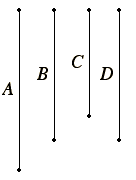# Proposition 17

If three straight lines are proportional, then the rectangle contained by the extremes equals the square on the mean; and, if the rectangle contained by the extremes equals the square on the mean, then the three straight lines are proportional.

Let the three straight lines A and B and C be proportional, so that A is to B as B is to C.

I say that the rectangle A by C equals the square on B.I.3

Make D equal to B.

Then, since A is to B as B is to C, and B equals D, therefore A is to B as D is to C.

VI.16

But, if four straight lines are proportional, then the rectangle contained by the extremes equals the rectangle contained by the means.

Therefore the rectangle A by C equals the rectangle B by D. But the rectangle B by D is the square on B, for B equals D, therefore the rectangle A by C equals the square on B.

Next, let the rectangle A by C equal the square on B.

I say that A is to B as B is to C.

With the same construction, since the rectangle A by C equals the square on B, while the square on B is the rectangle B by D, for B equals D, therefore the rectangle A by C equals the rectangle B by D.

VI.16

But, if the rectangle contained by the extremes equals that contained by the means, then the four straight lines are proportional.

Therefore A is to B as D is to C.

But B equals D, therefore A is to B as B is to C.

Therefore, if three straight lines are proportional, then the rectangle contained by the extremes equals the square on the mean; and, if the rectangle contained by the extremes equals the square on the mean, then the three straight lines are proportional.

Q.E.D.

## Guide

This is obviously a special case of the previous proposition. It is used very frequently in Books X and XIII.